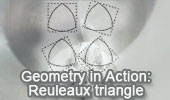# Online Geometry: Equilateral Triangles, Theorems and Problems - Page 3Proposed Problem 366.
Scalene triangle, Circumcircle, Angles, 60 Degrees, Equilateral triangle.

Proposed Problem 365.
Circular Sector of 60 degrees, Midpoints, Perpendicular, Congruence.

Proposed Problem 326.
Equilateral triangle, Semicircle, Equal arcs.

Proposed Problem 248.
Napoleon's Theorem III. Inner and outer Napoleon triangles, Area.

Proposed Problem 247.
Napoleon's Theorem II. Internal Equilateral triangles. Inner Napoleon triangle.

Proposed Problem 246.
Napoleon's Theorem I. External Equilateral triangles.

Morley's Triangle & Center: with interactive animation and manipulation.

Proposed Problem 262.
Regular Hexagon inscribed in a circle, sum of distances.

Proposed Problem 260.
Equilateral Triangle, Incircle, Tangency Points, Vertices, Distances, Squares.

Proposed Problem 259.
Equilateral Triangle, Incircle, Tangency Points, Side, Distances, Squares.

Proposed Problem 258.
Equilateral Triangle, Circumcircle, Point, Vertices, Side, Distances, Squares.

Proposed Problem 257.
Equilateral Triangle, Circumcircle, Point, Vertices, Side, Distances, Squares.

Proposed Problem 256.
Equilateral Triangle, Circumcircle, Point, Vertices, Distances.

Proposed Problem 245. Parallelogram with Equilateral triangles

Proposed Problem 243. Triangle with Equilateral triangles, Parallelogram.

Proposed Problem 242. Triangle with Equilateral triangles, Parallelogram.

Proposed Problem 241. Triangle with Equilateral triangles, Congruence.

Proposed Problem 240. Triangle with Equilateral triangles, Parallelogram.

Proposed Problem 225. Viviani's Theorem Extension, Regular Polygon, Apothem, Distance.

Proposed Problem 222. Viviani's theorem, Equilateral triangle, Exterior point, Distances.

Proposed Problem 221. Viviani's theorem, Equilateral triangle, Interior point, Distances.

Proposed Problem 212. 120 Degree Triangle, Equilateral triangles, Areas.

Proposed Problem 211. 60 Degree Triangle, Equilateral triangles, Areas.Geometry in Action. Reuleaux's rotor: How Round is your Circle? The Reuleaux triangle is a constant width curve based on an equilateral triangle.

Proposed Problem 132.
Triangle, 60 degree, Orthocenter, Congruence, Midpoint.

Proposed Problem 103.Equilateral Triangle Area, Interior Point, Heron's Formula.

Proposed Problem 102.Equilateral Triangle Area, Interior Point.

Proposed Problem 101.Equilateral Triangle, Pythagorean Theorem, Angles.

Machu Picchu and Sierpinski Triangle. The canonical Sierpinski triangle uses an equilateral triangle with a base parallel to the horizontal axis.

Go to Page: Previous | 1 | 2 | 3 | 4 | 5 | Next

 Home | Search | Geometry | Triangles | Equilateral Triangle | Post a comment | Email | By Antonio Gutierrez